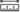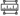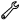# Measure scenes

The measure tools allow you to measure distances between two points and calculate areas in your scene. When you click the Measure distance toolor Measure area tool, horizontal laser lines are projected on the terrain and objects in the scene. This shows the vertical height of the pointer in the scene as you measure. It is helpful to see the heights of objects relative to other objects and the terrain. For example, you can hover over a smaller building and see that height highlighted relative to another part of the terrain.

## Measure distance

Use the Measure distancetool to calculate the distance between two points in a scene. Scene Viewerl labels the direct, horizontal, and vertical distance lines in the scene and displays the values in the panel.

• Direct—Distance between two points
• Horizontal—Horizontal distance between two points
• Vertical—Vertical distance between two points

While you are measuring, a second laser line indicates where the vertical plane along the checkered line intersects the terrain in all directions, such as with buildings, bridges, and the ground.

To measure distance, do the following:

1. Click Analyzeto open the measure tools.
2. Click Measure distance.
3. Click in the scene to start measuring.
4. Click to set the endpoint.
5. Click New Measurement to start a new measurement.

When the distance between the points is greater than 100 kilometers, a circular laser line appears, indicating that Scene Viewer has switched to geodesic mode. In geodesic mode, Scene Viewer calculates only the horizontal and vertical distances, taking into consideration the curvature of the earth (that is, ellipsoid-based geodesic distance). The Direct distance option is unavailable.

## Measure area

Use the Measure area toolto calculate the area of a polygon you draw. While measuring, the Measure area tool labels the current segment length and the total length of the path in your scene. Once you close the path, a polygon is created with labeled values for the area and perimeter. These values also appear in the panel.

• Area—Area of the polygon
• Perimeter—Perimeter length of the polygon

To measure area, do the following:

1. Click Measure area.
2. Click in the scene to start adding points to the polygon.
3. Double-click to close the path and calculate the polygon area. Alternatively, click the starting point again to close the path.
4. Click New Measurement to start a new measurement.

When the polygon perimeter is greater than 100 kilometers, Scene Viewer switches to geodesic mode. In geodesic mode, Scene Viewer calculates the values, taking into consideration the curvature of the earth (that is, ellipsoid-based geodesic values).

To adjust either the Measure distanceor Measure areameasurement, hover over a point and drag. As you drag points, Scene Viewer displays the adjusted values in the scene and panel. You can change the unit of measure under Unit.